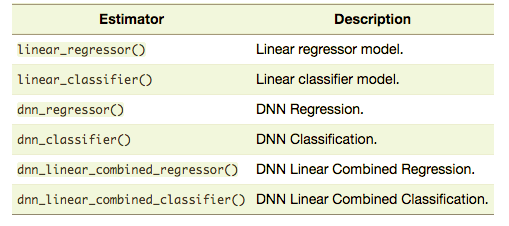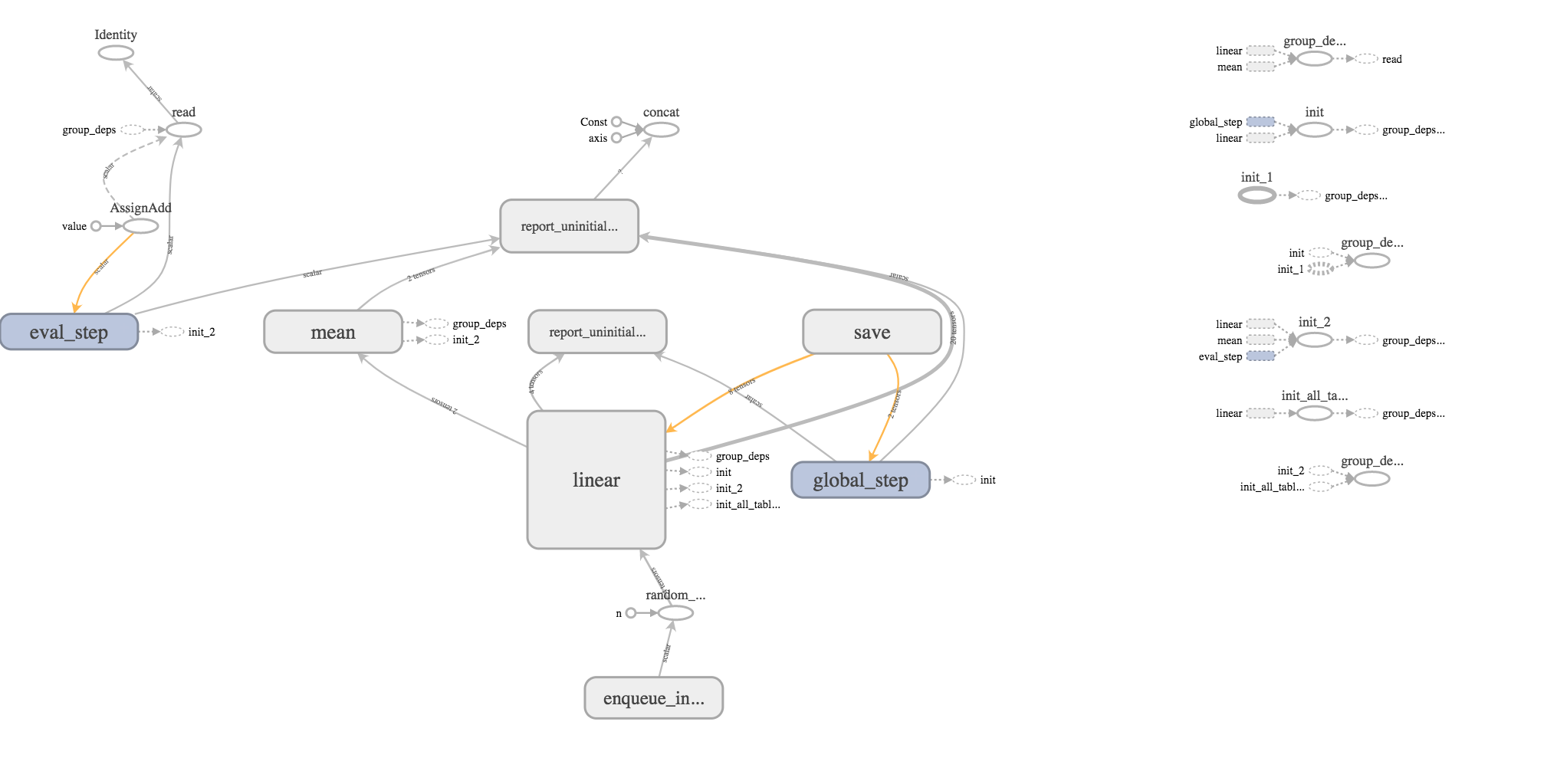# Fitting a TensorFlow Linear Classifier with tfestimators

by Joseph Rickert & Edgar Ruiz

In a recent post, I mentioned three avenues for working with TensorFlow from R:
* The keras package, which uses the Keras API for building scaleable, deep learning models * The tfestimators package, which wraps Google’s Estimators API for fitting models with pre-built estimators
* The tensorflow package, which provides an interface to Google’s low-level TensorFlow API

In this post, Edgar and I use the linear_classifier() function, one of six pre-built models currently in the tfestimators package, to train a linear classifier using data from the titanic package.library(tfestimators)
library(tensorflow)
library(tidyverse)
library(titanic)

The titanic_train data set contains 12 fields of information on 891 passengers from the Titanic. First, we load the data, split it into training and test sets, and have a look at it.

titanic_set <- titanic_train %>% filter(!is.na(Age))

# Split the data into training and test data sets
indices <- sample(1:nrow(titanic_set), size = 0.80 * nrow(titanic_set))
train <- titanic_set[indices, ]
test  <- titanic_set[-indices, ]

glimpse(titanic_set)
## Observations: 714
## Variables: 12
## $PassengerId <int> 1, 2, 3, 4, 5, 7, 8, 9, 10, 11, 12, 13, 14, 15, 16... ##$ Survived    <int> 0, 1, 1, 1, 0, 0, 0, 1, 1, 1, 1, 0, 0, 0, 1, 0, 0,...
## $Pclass <int> 3, 1, 3, 1, 3, 1, 3, 3, 2, 3, 1, 3, 3, 3, 2, 3, 3,... ##$ Name        <chr> "Braund, Mr. Owen Harris", "Cumings, Mrs. John Bra...
## $Sex <chr> "male", "female", "female", "female", "male", "mal... ##$ Age         <dbl> 22, 38, 26, 35, 35, 54, 2, 27, 14, 4, 58, 20, 39, ...
## $SibSp <int> 1, 1, 0, 1, 0, 0, 3, 0, 1, 1, 0, 0, 1, 0, 0, 4, 1,... ##$ Parch       <int> 0, 0, 0, 0, 0, 0, 1, 2, 0, 1, 0, 0, 5, 0, 0, 1, 0,...
## $Ticket <chr> "A/5 21171", "PC 17599", "STON/O2. 3101282", "1138... ##$ Fare        <dbl> 7.2500, 71.2833, 7.9250, 53.1000, 8.0500, 51.8625,...
## $Cabin <chr> "", "C85", "", "C123", "", "E46", "", "", "", "G6"... ##$ Embarked    <chr> "S", "C", "S", "S", "S", "S", "S", "S", "C", "S", ...

Notice that both Sex and Embarked are character variables. We would like to make both of these categorical variables for the analysis. We can do this “on the fly” by using thetfestimators::feature_columns() function to get the data into the shape expected for an input Tensor. Category levels are set by passing a list to the vocabulary_list argument. The Pclass variable is passed as a numeric feature, so no further action is required.

cols <- feature_columns(
column_categorical_with_vocabulary_list("Sex", vocabulary_list =
list("male", "female")),
column_categorical_with_vocabulary_list("Embarked", vocabulary_list =
list("S", "C", "Q", "")),
column_numeric("Pclass")
)

So far, no real processing has taken place. The data have not yet been evaluated by R or loaded into TensorFlow. Our first interaction with TensorFlow begins when we use the linear_classifier() function to build the TensorFlow model object for a linear model.

model <- linear_classifier(feature_columns = cols)

Now, we use the tfestimators::input_fn() to get the data into TensorFlow and define the model itself. The following helper function sets up the predictive variables and response variable for a model to predict survival from knowing a passenger’s sex, ticket class, and port of embarkation.

titanic_input_fn <- function(data) {
input_fn(data,
features = c("Sex", "Pclass", "Embarked"),
response = "Survived")
}

tfestimators::train() uses the helper function to fit and train the model on the training set constructed above.

train(model, titanic_input_fn(train))

The tensorflow::evaluate() function evaluates the model’s performance.

model_eval <- evaluate(model, titanic_input_fn(test))
glimpse(model_eval)  
## Observations: 1
## Variables: 9
## $loss <dbl> 40.2544 ##$ accuracy_baseline    <dbl> 0.5874126
## $global_step <dbl> 5 ##$ auc                  <dbl> 0.8096247
## $prediction/mean <dbl> 0.3557937 ##$ label/mean         <dbl> 0.4125874
## $average_loss <dbl> 0.5629987 ##$ auc_precision_recall <dbl> 0.8102072
## $accuracy <dbl> 0.7132867 It’s not a great model, by any means, but an AUC of 0.85 isn’t bad for a first try. We will use R’s familiar predict() function to make some predictions with the test data set. Notice that this data needs to be wrapped in the titanic_input_fn() just like we did for the training data above. model_predict <- predict(model, titanic_input_fn(test)) The following code unpacks the list containing the prediction results. res <- data.frame(matrix(unlist(model_predict[]),ncol=2,byrow=TRUE), unlist(model_predict[]), unlist(model_predict[]), unlist(model_predict[]), unlist(model_predict[])) names(res) <- c("Prob Survive", "Prob Perish",names(model_predict)[2:5]) options(digits=3) head(res) ## Prob Survive Prob Perish logits classes class_ids logistic ## 1 0.380 0.620 0.4899 1 1 0.620 ## 2 0.509 0.491 -0.0373 0 0 0.491 ## 3 0.380 0.620 0.4899 1 1 0.620 ## 4 0.509 0.491 -0.0373 0 0 0.491 ## 5 0.781 0.219 -1.2697 0 0 0.219 ## 6 0.735 0.265 -1.0180 0 0 0.265 Before finishing up, we note that TensorFlow writes quite a bit of information to disk: list.files(model$estimator$model_dir) ##  "checkpoint" "eval" ##  "graph.pbtxt" "logs" ##  "model.ckpt-1.data-00000-of-00001" "model.ckpt-1.index" ##  "model.ckpt-1.meta" "model.ckpt-5.data-00000-of-00001" ##  "model.ckpt-5.index" "model.ckpt-5.meta" Finally, we use the TensorBoard visualization tool to look at the data flow graph and other aspects of the model.To see all of this, point your browser to address returned by the following command. tensorboard(model$estimator\$model_dir, action="start") 
## Started TensorBoard at http://127.0.0.1:5503